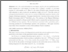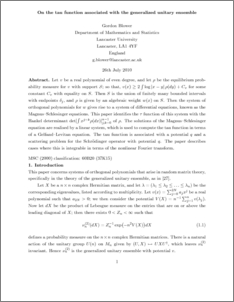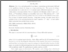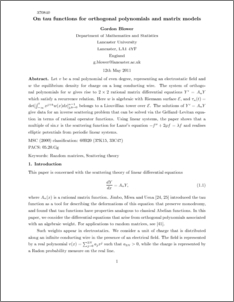# On tau functions for orthogonal polynomials and matrix models.

Blower, Gordon (2011) On tau functions for orthogonal polynomials and matrix models. Journal of Physics A: Mathematical and Theoretical, 44 (28). pp. 1-31. ISSN 1751-8113Preview
PDF (TAU.pdf)
TAU.pdfPreview
PDF
taushortrevised.pdf - Submitted Version

## Abstract

Let v be a real polynomial of even degree, and let \rho be the equilibrium probability measure for v with support S; to that v(x) greeater than or equal to \int 2log |x-y| \rho (dy) +C for some constant C with equality on S. THen S is the union of finitely many boundd intervals with endpoints \delta_j and \rho is given by an algebraic weight w(x) on S. Then the system of orthogonal polynomials for w gives rise to a system of differential equations, known as the Schlesinger equations. This paper identifies the tau function of this system with the Hankel determinant \det [\int x^{j+k}\rho (dx)]. The solutions of the Magnus--Schlesinger equation are realised by a linear system, which is used to compute the tau functions in terms of a Gelfand--Levitan equation. The tau function is associated with a potential q and a scattering problem for the Schrodinger equation with potential q. The paper describes cases where this is integrable in terms of the nonlinear Fourier transform.

Item Type:
Journal Article
Journal or Publication Title:
Journal of Physics A: Mathematical and Theoretical
Uncontrolled Keywords:
/dk/atira/pure/researchoutput/libraryofcongress/qa
Subjects:
Departments:
ID Code:
40861
Deposited By:
Deposited On:
13 Jun 2011 10:33
Refereed?:
Yes
Published?:
Published